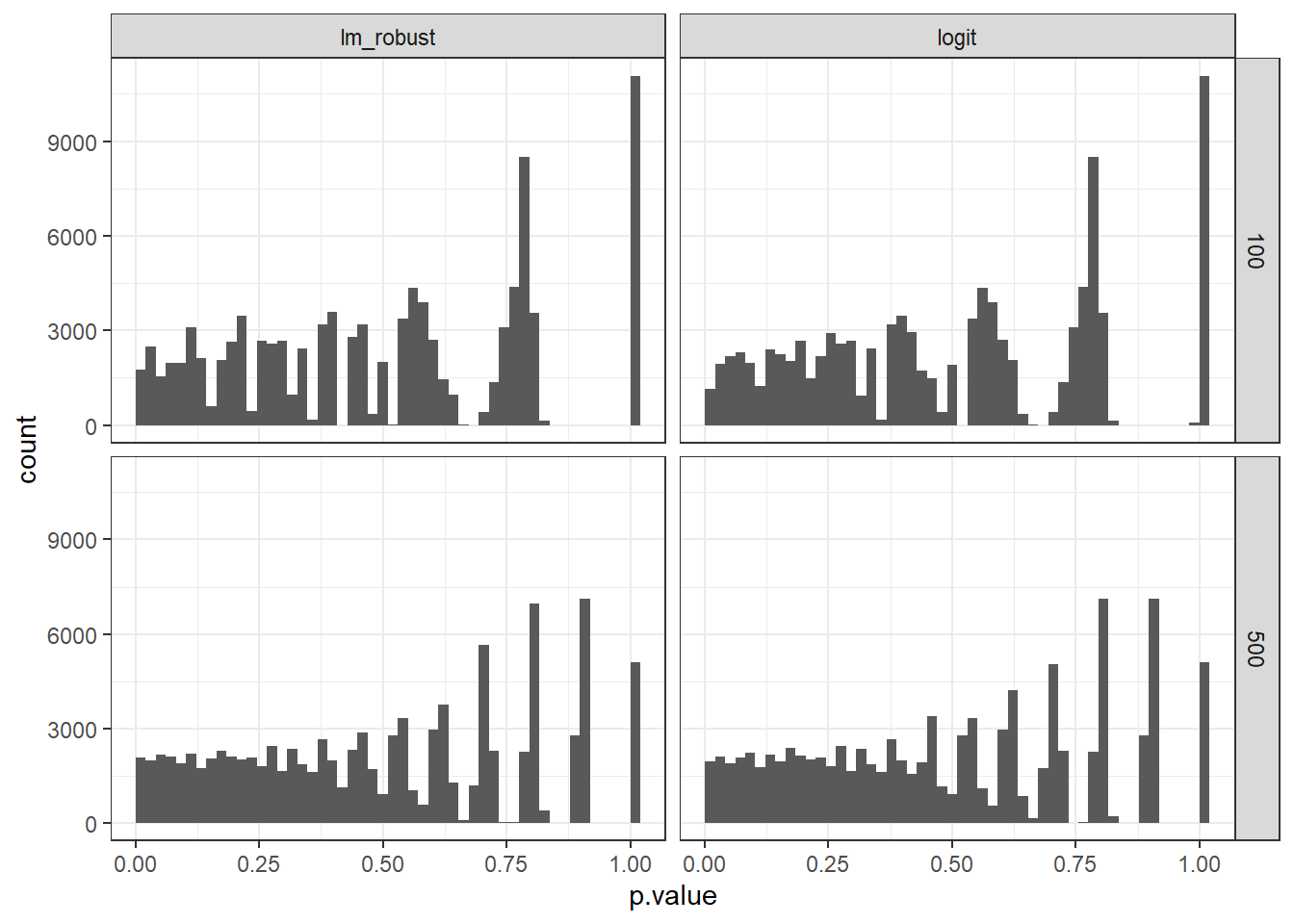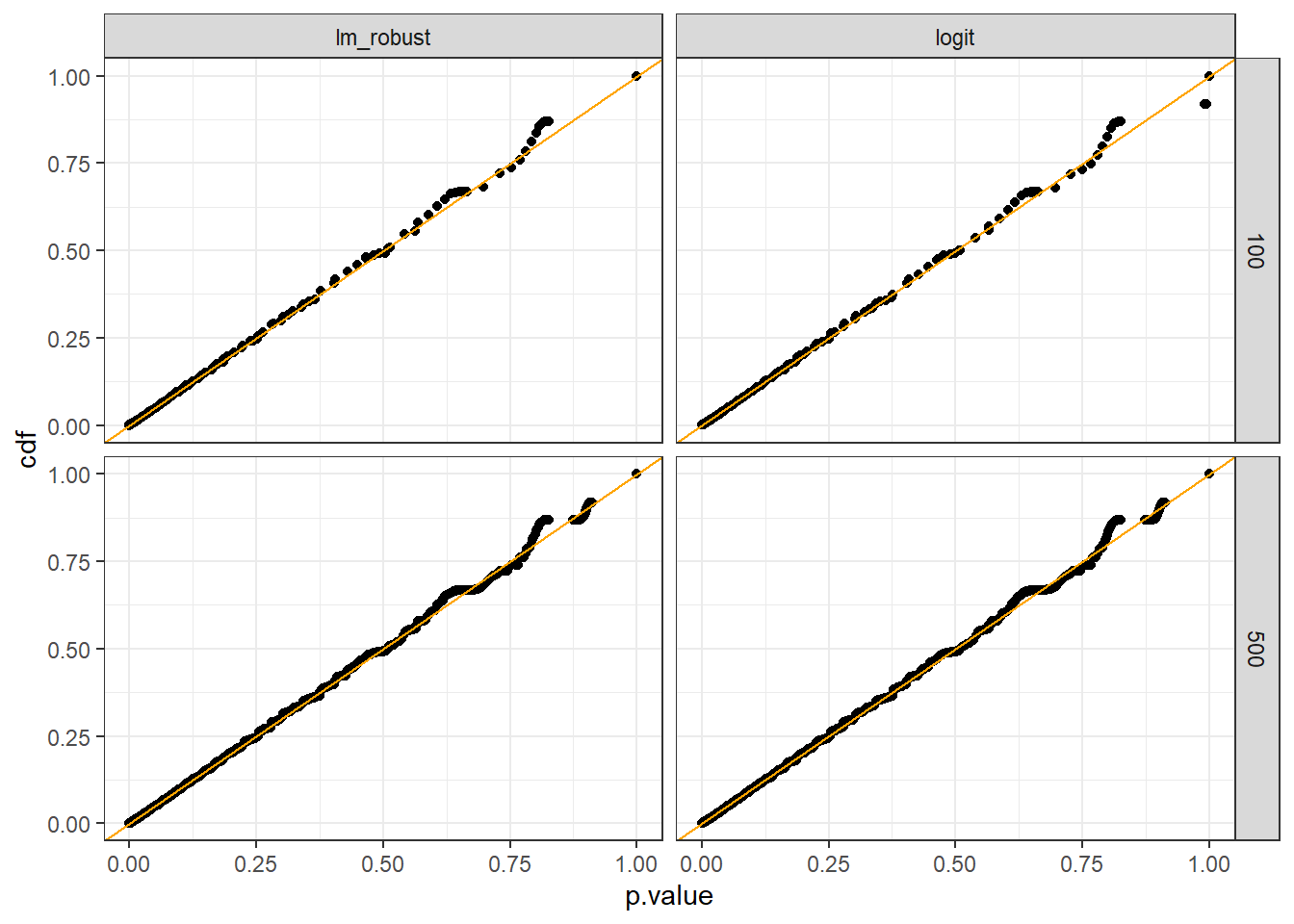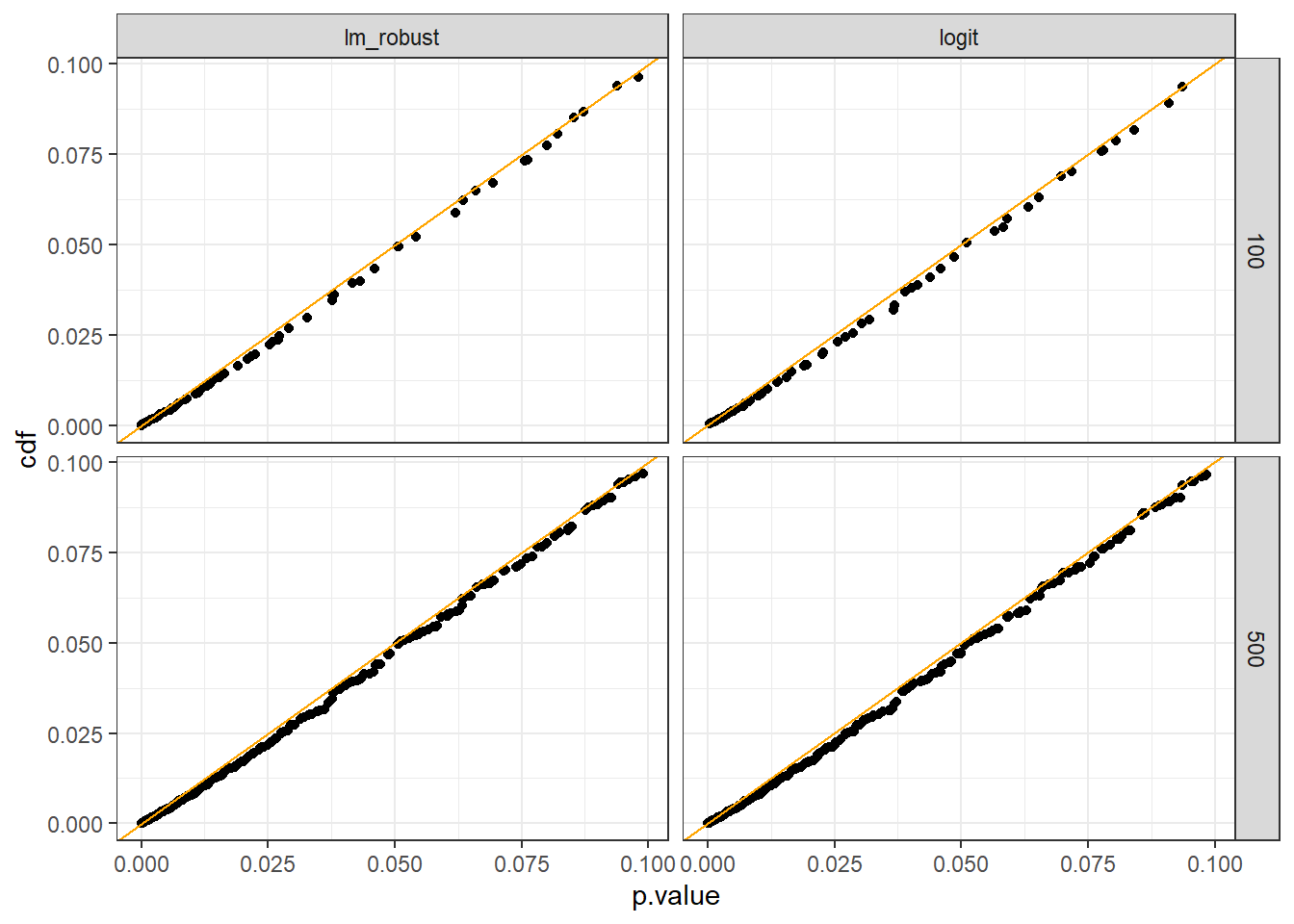# 1 Overview

Last week in class we discussed the idea that $$p$$ values are uniformly distributed under the null. Coincidentally, the same day Richard McElreath put out an interesting thread saying that they are not, not even in theory. https://twitter.com/rlmcelreath/status/1677322772719054849 1

McElreath showed an example where the distribution seemed very far from uniform for a pretty standard application: an experiment with a binary $$X$$ and $$Y$$ and a null of no effect for any unit (so his example presupposed a sharp null).

## 1.1 Concepts

First a conceptual clarification on what $$p$$ values are. We can distinguish between the real, or actual $$p$$ values and the estimated, or nominal, $$p$$-values. The real (or actual) $$p$$ value reports the probability of seeing such a large test statistic (e.g. estimate) under some null, for instance the null of no effect. The estimated (or nominal) $$p$$ value is what gets reported in papers and it is an estimate of the real $$p$$ value.

## 1.2 What’s at stake

If the $$p$$ values are far off in this simple case then we really have a problem. (Of course we do have multiple problems with $$p$$s, but the issue here is whether they are reliable on their own merits.)

Why’s that? Because we get two distinct things from the claim that $$p$$ values are uniform over $$[0,1]$$.

• A. First we get from this the claim that the probability of seeing a $$p$$ value less than or equal to $$\alpha$$ under the null is itself $$\alpha$$. So the probability of seeing a $$p$$ value of 0.05 or less is 0.05. (“Validity” definitions have a weak inequality: $$\Pr(p \leq \alpha)) \leq \alpha$$.)
• B. Second we have an expectation of what the distribution of $$p$$ values should look like if indeed the null is true – for example for balance tests (should you do them) for baseline covariates after randomization. This feature is sometimes used to assess the risk of fraud. To wit: https://twitter.com/GidMK/status/1656228772633522177

McElreaths’s critique could apply to either the real or the estimated $$p$$-values and threaten both claim B and, perhaps, claim A.

To explore further I took a bit of a deep dive into McElreath’s example to understand better what’s going on there. I ran the same simulation but increased the number of simulations, letting $$N$$ and the estimation method vary, and also calculating the estimated (“nominal” $$p$$ values) as well as the correct $$p$$ values (analytically and via simulation) as well as the exact randomization inference $$p$$ values for nulls for a collection of sample average treatment effects.

Full declaration and simulations are below but the headlines are:

• it is unambiguous that both actual and nominal $$p$$ values can fail with respect to $$B$$: you can see this directly from McElreaths’s histogram for the nominal $$p$$ values, but it is also true for the real $$p$$ values. We saw examples of this already in the randomization inference applications. Thus the distribution of $$p$$ values will obviously not be uniform over [0,1]. This is a challenges for claim B for both real and nominal $$p$$ values.
• real $$p$$ values should not fail with respect to $$A$$. To see why, imagine the sampling distribution of the estimates—all the estimates given our data and answer strategy. Then the $$p$$ value associated with some estimate $$b$$ that you might get is the probability mass at or beyond $$b$$ and $$-b$$. Any test statistic beyond $$b$$ that might arise will produce a lower $$p$$ value. So if test statistic $$x$$ produces value $$p$$ then the probability of $$p$$ or lower is that probability of a statistic as large or larger than $$x$$ in absolute value, which is exactly what $$p$$ reports.
• even if $$B$$ fails it is still possible that $$A$$ holds for nominal $$p$$ values. For instance when we generate $$p$$ values through randomization inference we are guaranteed that this property will hold when the distribution is taken with respect to the randomization. In fact when we calculate the exact distribution (or look with a large number of simulations) it looks like this property does hold well for low values of $$\alpha$$; things go off somewhat at the high end. However the $$\alpha$$s used in tests are typically taken from the low end, e.g. 0.01, 0.05, 0.1, where as far as I can see things really do look OK.

## 1.4 Secondary results

A couple of other things of note.

• The issue here doesn’t seem to have much to do with OLS or logit; these produce essentially the same distributions of $$p$$ values (though with some interesting exceptions).
• The real $$p$$ values have an even smaller domain that the domain from the estimates from OLS and logit. This is because these are calculated using the sampling distribution of estimates only whereas the estimated (nominal) $$p$$ values also use information of the estimated variance of the sampling distribution, which varies across runs. Thus the true distribution of (actual $$p$$ values) faces challenge $$A$$ but not challenge $$B$$.
• The randomization inference $$p$$ values have property B (are valid) conditional on the distribution of $$Y$$—these are constructed to be so; but they do not have this feature across distributions of $$Y$$. This is because they target the sample average effect. Nevertheless it means that if we see multiple $$p$$ values generated via randomization inference for different outcomes (for instance) we should not expect the $$p$$ values to be uniformly distributed.

## 1.5 And also

• These notes show how to do this kind of simulation in DeclareDesign along with a trick to use multistage simulation to calculate randomization inference $$p$$ values.
• The code is written so that it is easy to alter the key features of the design: the $$N$$ and the probability $$Y = 1$$.

# 2 Simulation approach

I start with a simulation approach since although it’s not necessary for the current example it is more flexible in general.

## 2.1 Basic Declaration

The simulations are done using DeclareDesign. The design is very simple:

N <- 100

design <-
declare_model(N = N, X = rep(0:1, N/2), Y = rbinom(N, 1, 0.85)) +
declare_estimator(Y ~ X, .method = glm, family = binomial(), label = "logit") +
declare_estimator(Y ~ X, .method = lm_robust, label = "lm_robust") 

If you simulate this design and plot the $$p$$ values you get essentially the same graph as in the post. And you get essentially the same graph whether you use lm_robust or probit.

Because I am doing a very large number of simulations I am going to save the simulation object and read it back in (run turns the simulations on and off).

if(run)
design |>
redesign(N = c(100, 500)) |>   # Vary N
simulate_design(sims = 100000) |> write_rds("sims_100_500.rds")

mutate(cdf = cume_dist(round(p.value, 4)))

simulations |>
ggplot(aes(p.value)) + geom_histogram(bins = 50, boundary = 0) + facet_grid(N~estimator)Distribution of nominal p values

Note that I coarsened the p.values slightly—rounding to 4 decimal places—to make the cdf easier to read by being unresponsive to extremely tiny differences in estimate $$p$$ values.

If you plot the cdf you see it does pretty well at the low end. Things go wacky at the high. Though in practice people really use $$p$$ values at the low end.

simulations |> ggplot(aes(p.value, cdf)) + geom_point() +
facet_grid(N~estimator) +
geom_abline(intercept = 0, slope = 1, color = "orange")Let’s zoom in to $$p$$ values in the range $$(0, .1]$$:

simulations |> filter(p.value <= .1) |>
ggplot(aes(p.value, cdf)) + geom_point() +
facet_grid(N~estimator) +
geom_abline(intercept = 0, slope = 1, color = "orange")On visual inspection here the fit is very good. Things are quite good too when you look at a set of specific values:

simulations |> filter(N == 100 & estimator == "lm_robust") |> pull(p.value) |>
quantile(probs = c(.01, .02, .03, .04, .05))|> round(3)
##    1%    2%    3%    4%    5%
## 0.011 0.021 0.029 0.038 0.051

Fit as a regression the $$R^2$$ would be 99.8% (lm(cdf ~ p.value, data = simulations)).

Things are still better with a larger $$N$$ which allows for a fuller support for the $$p$$-values.

simulations |> filter(N == 500 & estimator == "lm_robust") |> pull(p.value) |>
quantile(probs = c(.01, .02, .03, .04, .05))|> round(3)
##    1%    2%    3%    4%    5%
## 0.010 0.020 0.030 0.039 0.049

## 2.2 Comparison with the real $$p$$ values

How close are the “nominal” $$p$$-values estimated here to the “real” $$p$$’s?

Recall the real $$p$$ values report the probability of getting an estimate as large or larger than what we get under the null hypothesis. Since we hvae lots of data generated under the null hypothesis we can use the simulations we already have to calculate these real $$p$$ values up to simulation error.

Let’s do it here for the lm case.

true_p <-
simulations |> filter(estimator == "lm_robust") |>
group_by(N) |>
mutate(p = 1-percent_rank(c(10000, abs(round(estimate, 5))))[-1]) |>
ungroup() 

See again that the modal p value is not 1 but below 1, reflecting the case with an imbalance of 1 between treatment and control.

true_p |> ggplot(aes(p)) + geom_histogram(boundary = 0) + facet_grid(~N)
## stat_bin() using bins = 30. Pick better value with binwidth.